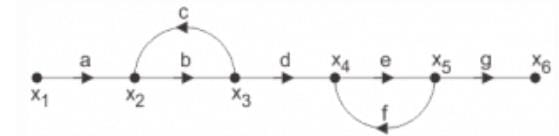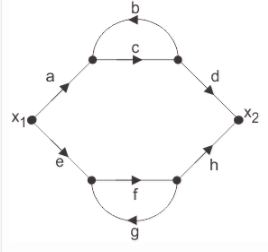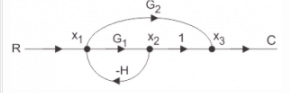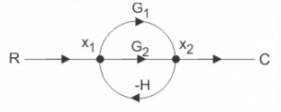# Test: Signal Flow Graphs

## 15 Questions MCQ Test GATE ECE (Electronics) 2023 Mock Test Series | Test: Signal Flow Graphs

Description
Attempt Test: Signal Flow Graphs | 15 questions in 10 minutes | Mock test for Electrical Engineering (EE) preparation | Free important questions MCQ to study GATE ECE (Electronics) 2023 Mock Test Series for Electrical Engineering (EE) Exam | Download free PDF with solutions
QUESTION: 1

### A signal flow graph is the graphical representation of the relationships between the variables of set linear algebraic equations.

Solution:

Explanation: By definition signal flow graphs are the graphical representation of the relationships between the variables of set linear algebraic equations.

QUESTION: 2

### A node having only outgoing branches.

Solution:

Explanation: Nodes are the point by which the branches are outgoing or ingoing and this can be input or output node and input node is the node having only outgoing branches.

QUESTION: 3

### Use mason’s gain formula to find the transfer function of the given signal flow graph:Solution:

Explanation: Using mason’s gain formula transfer function from signal flow graph can be calculated which relates the forward path gain to the various paths and loops.

QUESTION: 4

Use mason’s gain formula to find the transfer function of the following signal flow graph:Solution:

Explanation: Using mason’s gain formula transfer function from signal flow graph can be calculated which relates the forward path gain to the various paths and loops.

QUESTION: 5

Loop which do not possess any common node are said to be ___________ loops.

Solution:

Explanation: Loop is the part of the network in which the branch starts from the node and comes back to the same node and non touching loop must not have any node in common.

QUESTION: 6

Signal flow graphs:

Solution:

Explanation: Signal flow graphs are used to find the transfer function of control system by converting the block diagrams into signal flow graphs or directly but cannot be used for nonlinear systems.

QUESTION: 7

Signal flow graphs are reliable to find transfer function than block diagram reduction technique.

Solution:

Explanation: As one set technique and formula is used here but in block diagram technique various methods are involved which increases complexity.

QUESTION: 8

The relationship between an input and output variable of a signal flow graph is given by the net gain between the input and output node is known as the overall______________

Solution:

Explanation: The relationship between input and output variable of a signal flow graph is the overall gain of the system.

QUESTION: 9

Use mason’s gain formula to calculate the transfer function of given figure:Solution:

Explanation : Using mason’s gain formula transfer function from signal flow graph can be calculated which relates the forward path gain to the various paths and loops.

i.e. G1/1 + G2H

QUESTION: 10

Loop which do not possess any common node are said to be ___________ loops.

Solution:

Explanation: Loop is the part of the network in which the branch starts from the node and comes back to the same node and non touching loop must not have any node in common.

QUESTION: 11

Signal flow graphs:

Solution:

Explanation: Signal flow graphs are used to find the transfer function of control system by converting the block diagrams into signal flow graphs or directly but cannot be used for nonlinear systems.

QUESTION: 12

Signal flow graphs are reliable to find transfer function than block diagram reduction technique.

Solution:

Explanation: As one set technique and formula is used here but in block diagram technique various methods are involved which increases complexity.

QUESTION: 13

The relationship between an input and output variable of a signal flow graph is given by the net gain between the input and output node is known as the overall______________

Solution:

Explanation: The relationship between input and output variable of a signal flow graph is the overall gain of the system.

QUESTION: 14

Use mason’s gain formula to calculate the transfer function of given figure:Solution:

Explanation: Use mason’s gain formula to solve the signal flow graph and by using mason’s gain formula transfer function from signal flow graph can be calculated which relates the forward path gain to the various paths and loops.

QUESTION: 15

Use mason’s gain formula to find the transfer function of the given figure:Solution:

Explanation: Using mason’s gain formula transfer function from signal flow graph can be calculated which relates the forward path gain to the various paths and loops.Use Code STAYHOME200 and get INR 200 additional OFF Use Coupon Code